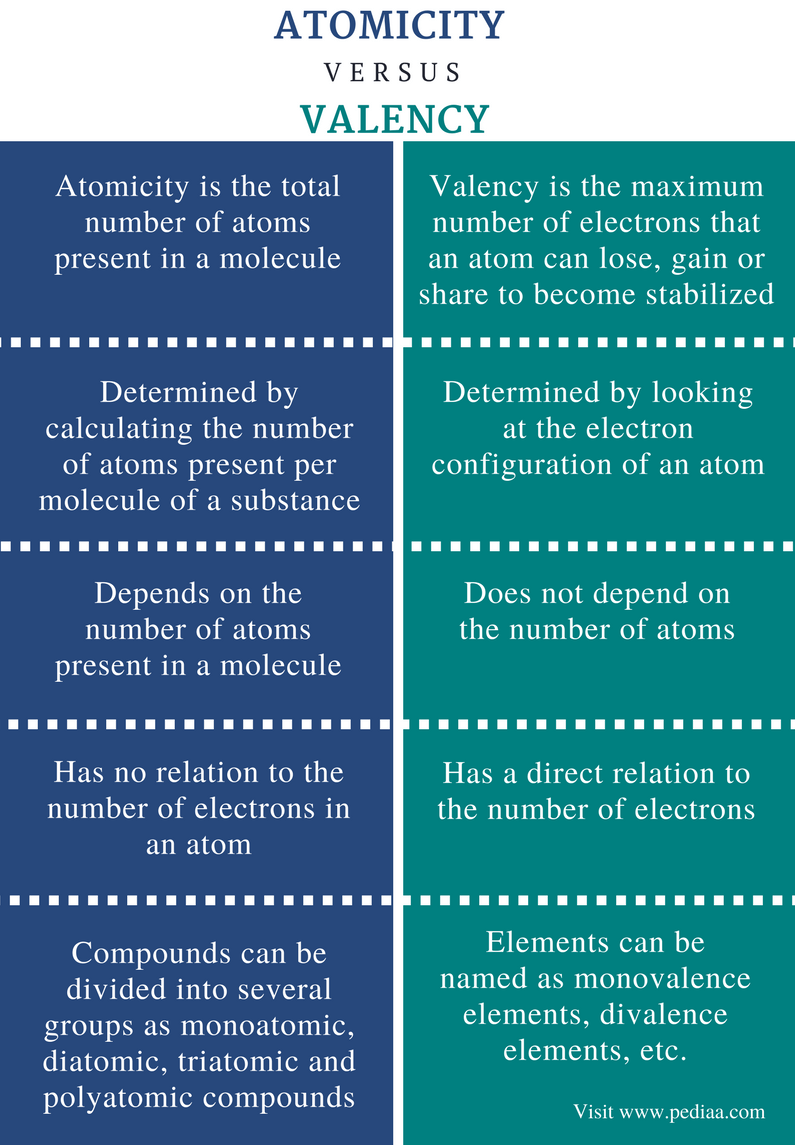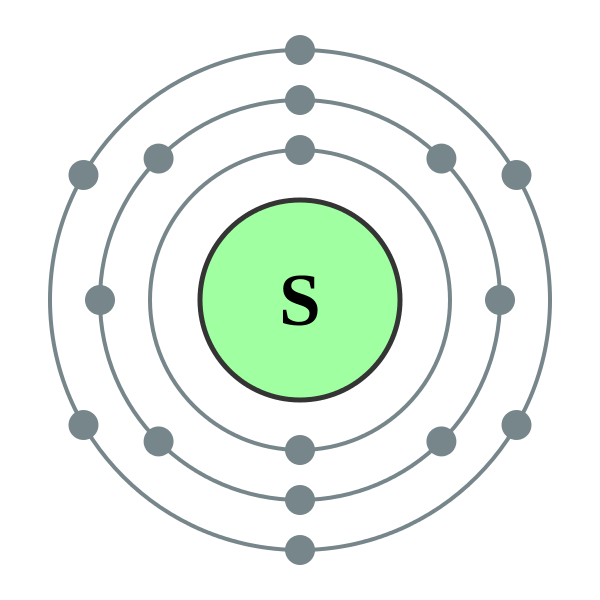# Difference Between Atomicity and Valency

## Main Difference – Atomicity vs Valency

Atomicity and valency are two chemical terms that are often used regarding atoms and molecules. Atomicity is the number of atoms present in a molecule. Valency is the maximum number of electrons that an atom has to lose, gain or share in order to get stabilized. Therefore these are two distinct properties of atoms and molecules. The main difference between atomicity and valency is that atomicity explains a molecular property whereas valency describes an elemental property.

### Key Areas Covered

1. What is Atomicity
– Definition, Types with Examples
2. What is Valency
– Definition, Explanation with an Example
3. What is the Difference Between Atomicity and Valency
– Comparison of Key Differences

Key Terms: Atomicity, Diatomic, Electron Configuration, Inert Gases, Monoatomic, Polyatomic, Sulfur, Valency## What is Atomicity

Atomicity is the total number of atoms present in a molecule. According to this definition, molecules can be divided into groups depending on the atomicity of molecules. For example, molecules can be monoatomic, diatomic, triatomic or polyatomic. Monoatomic compounds are composed of a single atom. For example, inert gases such as Helium (He), Argon (Ar), etc. are monoatomic compounds. Diatomic compounds are composed of two atoms per one molecule. Ex: oxygen gas (O2), nitrogen gas (N2), chlorine gas (Cl2), etc.

The symbol for atomicity is “a”. Therefore, for monoatomic compounds, a=1 and for diatomic compounds, a=2. For polyatomic compounds, the atomicity is a large value. This because the polyatomic molecules are composed of a large number of atoms.Figure 1: A Polyatomic Molecule

When the atomicity of compounds formed by the elements in the same group (in the periodic table) are considered, we can observe relationships in chemical and physical properties of those compounds. For example, all inert gases are monoatomic compounds which have similar chemical properties. Although some compounds are highly different from each other in their chemical and physical properties, they may belong to the same group of compounds depending on the atomicity of those compounds. For example, Cl2 is a gaseous compound whereas I2 is a solid at room temperature. But both these compounds are diatomic compounds when their atomicity values are considered.

## What is Valency

Valency can be defined as the maximum number of electrons that an atom can lose, gain or share in order to become stabilized. For metals and nonmetals, the octet rule describes the most stable form of an atom. It says that if the number of the outermost shell of an atom is completely filled with eight electrons, that configuration is stable. In other words, if the s and p sub-orbitals are completely filled, having ns2np6, it is stable. Naturally, noble gas atoms have this electron configuration. Therefore, other elements need to either lose, gain or share electrons in order to obey the octet rule. The maximum number of electrons involved in this stabilization process is called the valency of that atom.

Let us consider sulfur as an example. The valency of sulfur can be determined as below.Figure 2: Chemical Structure of Sulfur Atom

The number of electrons in the outermost orbital of sulfur is 6. In order become stabilized, the number of electrons in the outermost orbital should be 8 (according to the octet rule). Then, sulfur must gain or share 2 more electrons from outside. Therefore, the valency of sulfur is 2.

However, transition elements can have different valencies. This is because transition metals can be stabilized by removing different numbers of electrons.

## Difference Between Atomicity and Valency

### Definition

Atomicity: Atomicity is the total number of atoms present in a molecule.

Valency: Valency is the maximum number of electrons that an atom can lose, gain or share in order to become stabilized.

### Determination

Atomicity:  Atomicity can be determined by calculating the number of atoms present per molecule of a substance.

Valency:  Valency can be determined by looking at the electron configuration of an atom.

### Number of Atoms

Atomicity:  Atomicity depends on the number of atoms present in a molecule.

Valency: Valency does not depend on the number of atoms.

### Relation to Electron

Atomicity: Atomicity has no relation to the number of electrons in an atom.

Valency: Valency has a direct relation to the number of electrons.

### Different Types

Atomicity:  Compounds can be divided into several groups depending on the atomicity as monoatomic, diatomic, triatomic and polyatomic compounds.

Valency: Elements can be named as monovalence elements, divalence elements, etc. depending on the valency of the element.

### Conclusion

Atomicity is the number of atoms present in a molecule. Valency is the maximum number of electrons that an atom has to lose, gain or share in order to get stabilized. The main difference between atomicity and valency is that atomicity explains a molecular property whereas valency describes an elemental property.

##### References:

1. “CHEM-GUIDE.” Deduction of atomicity of elementary gas, chem-guide.blogspot.com/2010/03/Available here.
2. CHEMISTRY I: ATOMS AND MOLECULES. Available here.

##### Image Courtesy:

1. “Dodecaborane-3D-balls” Public Domain) via Commons Wikimedia
2. “Electron shell 016 Sulfur – no label” By commons:User:Pumbaa (original work by commons:User:Greg Robson)  (CC BY-SA 2.0 uk) via Commons Wikimedia